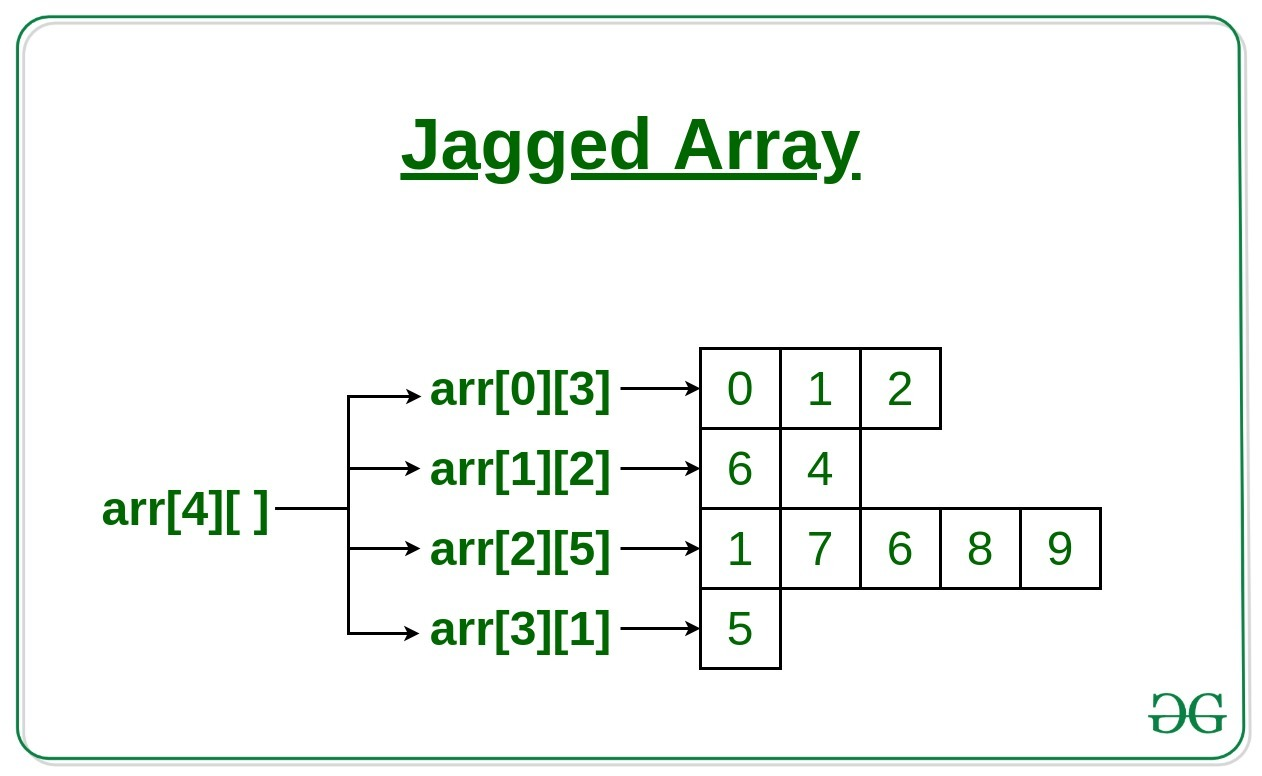# Jagged Array or Array of Arrays in C with Examples

Prerequisite: Arrays in C

Jagged array is array of arrays such that member arrays can be of different sizes, i.e., we can create a 2-D array but with a variable number of columns in each row. These type of arrays are also known as Jagged arrays.

Example:

```arr[][] = { {0, 1, 2},
{6, 4},
{1, 7, 6, 8, 9},
{5}
};
```Below are the methods to implement the jagged array in C:

1. Using array and a pointer (Static Jagged Array)
• First declare 1-D arrays with the number of rows you will need,
• The size of each array (array for the elements in the row) will be the number of columns (or elements) in the row,
• Then declare a 1-D array of pointers that will hold the addresses of the rows,
• The size of the 1-D array is the number of rows you want in the jagged array.

Below is the implementation of the above approach:
Example:

 `// C program to show the ` `// implementation of Jagged Arrays ` ` `  `#include ` `#include ` ` `  `int` `main() ` `{ ` ` `  `    ``int` `row0 = { 1, 2, 3, 4 }; ` `    ``int` `row1 = { 5, 6 }; ` ` `  `    ``int``* jagged = { row0, row1 }; ` ` `  `    ``// Array to hold the size of each row ` `    ``int` `Size = { 4, 2 }, k = 0; ` ` `  `    ``// To display elements of Jagged array ` `    ``for` `(``int` `i = 0; i < 2; i++) { ` ` `  `        ``// pointer to hold the address of the row ` `        ``int``* ptr = jagged[i]; ` ` `  `        ``for` `(``int` `j = 0; j < Size[k]; j++) { ` `            ``printf``(``"%d "``, *ptr); ` ` `  `            ``// move the pointer to the ` `            ``// next element in the row ` `            ``ptr++; ` `        ``} ` ` `  `        ``printf``(``"\n"``); ` `        ``k++; ` ` `  `        ``// move the pointer to the next row ` `        ``jagged[i]++; ` `    ``} ` ` `  `    ``return` `0; ` `} `

Output:

```1 2 3 4
5 6
```
2. Using an array of pointer (Dynamic Jagged Array)
• Declare an array of pointers (jagged array),
• The size of this array will be the number of rows required in the Jagged array
• Then for each pointer in the array allocate memory for the number of elements you want in this row.

Below is the implementation of the above approach:
Example:

 `// C program to show the ` `// implementation of Jagged Arrays ` ` `  `#include ` `#include ` ` `  `int` `main() ` `{ ` `    ``// 2 Rows ` `    ``int``* jagged; ` ` `  `    ``// Allocate memory for elements in row 0 ` `    ``jagged = ``malloc``(``sizeof``(``int``) * 1); ` ` `  `    ``// Allocate memory for elements in row 1 ` `    ``jagged = ``malloc``(``sizeof``(``int``) * 3); ` ` `  `    ``// Array to hold the size of each row ` `    ``int` `Size = { 1, 3 }, k = 0, number = 100; ` ` `  `    ``// User enters the numbers ` `    ``for` `(``int` `i = 0; i < 2; i++) { ` ` `  `        ``int``* p = jagged[i]; ` ` `  `        ``for` `(``int` `j = 0; j < Size[k]; j++) { ` `            ``*p = number++; ` ` `  `            ``// move the pointer ` `            ``p++; ` `        ``} ` `        ``k++; ` `    ``} ` ` `  `    ``k = 0; ` ` `  `    ``// Display elements in Jagged array ` `    ``for` `(``int` `i = 0; i < 2; i++) { ` ` `  `        ``int``* p = jagged[i]; ` `        ``for` `(``int` `j = 0; j < Size[k]; j++) { ` ` `  `            ``printf``(``"%d "``, *p); ` `            ``// move the pointer to the next element ` `            ``p++; ` `        ``} ` `        ``printf``(``"\n"``); ` `        ``k++; ` `        ``// move the pointer to the next row ` `        ``jagged[i]++; ` `    ``} ` ` `  `    ``return` `0; ` `} `

Output:

```100
101 102 103
```

My Personal Notes arrow_drop_upCheck out this Author's contributed articles.

If you like GeeksforGeeks and would like to contribute, you can also write an article using contribute.geeksforgeeks.org or mail your article to contribute@geeksforgeeks.org. See your article appearing on the GeeksforGeeks main page and help other Geeks.

Please Improve this article if you find anything incorrect by clicking on the "Improve Article" button below.